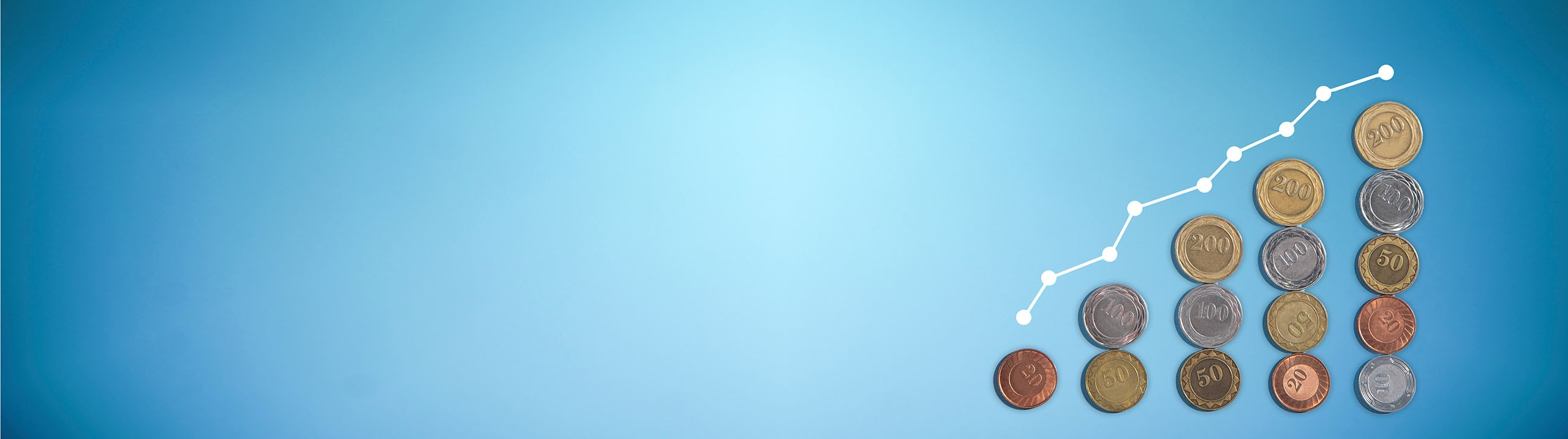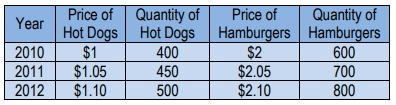## Macroeconomics

Learn the toughest concepts covered in your Macroeconomics class with step-by-step video tutorials and practice problems.

Gross Domestic Product (GDP) and Consumer Price Index (CPI)

# Nominal GDP and Real GDP

1
concept

## Nominal GDP and Real GDP9m
Play a video:
2
concept

## Inflation and the GDP Deflator3m
Play a video:
Okay so one use for the nominal GDP and real GDP why we might monitor both of them is to monitor inflation. So inflation. I'm sure you've heard of this word. It's where prices are rising in an economy. Right? So where price levels are generally rising from one year to the next, that's us going through inflation. So we can use nominal GDP and Real GDP to monitor inflation. Okay. And the general price levels. So what we're gonna use is what's called the GDP deflator. And the GDP deflator helps us calculate the inflation rate. So we've got our calculation for both GDP deflator and inflation right here notice GDP deflator, we're gonna have to calculate the nominal GDP in that year and divided by the real GDP in that year. So you can assume when we have inflation that the nominal GDP is going to be higher than the real GDP right? Because the higher prices leads to higher nominal GDP in the current year using current year prices. Um so we'll end up with this number above 100. Right, because this will the numerator will be bigger than the denominator and we'll have this uh measure that shows a higher number here. So we calculate the inflation rate by finding the change in the GDP deflator from one year to the next. So I know it's kind of long year GDP deflator in year two minus GDP deflator in year one over GDP deflator in year one. Well this is just a percentage change calculation. This is just percentage change calculation. Okay. And anytime we do a percentage change calculation it's very easy to do these, you just have to remember a very simple formula where we do new minus old over old. Okay, so to find the percentage change in any situation, we just do the new number minus the old number divided by the old number. So that's the way you want to remember percentage change. New minus old over old and that's exactly what's going on here. New GDP deflator in year two minus old GDP deflator in year one divided by old year one. Okay, So one thing to note about the GDP deflator is that in the base year it will always equal 100. Why is that? Because if we're talking about the base year, well what price level is used in the base here, phenomenal. The base year price. Right, because we're in the base here, the current year is the base here. So the base year price is used here, a nominal. The base here prices used in the real GDP. So we just get the same number in the numerator and denominator times 100 gives us 100. So it's going to equal 100 that's because nominal GDP and real GDP are equal to each other in the base here. Okay, so this isn't too tough. It's just an application of using nominal and real GDP, we're gonna go into a lot of detail of inflation and the implications of inflation in the economy in future videos. But now let's do a little bit of calculations with those numbers. Alright, let's pause here and do some practice.
3
Problem

The United States of Barbeque produces two goods:Hot Dogs and Hamburgers. Use the following information to calculate the GDP Deflator for 2012, assuming the base year is 2010.4
Problem

Using the information above, calculate the inflation rate for 2012 in the United States of Barbeque.